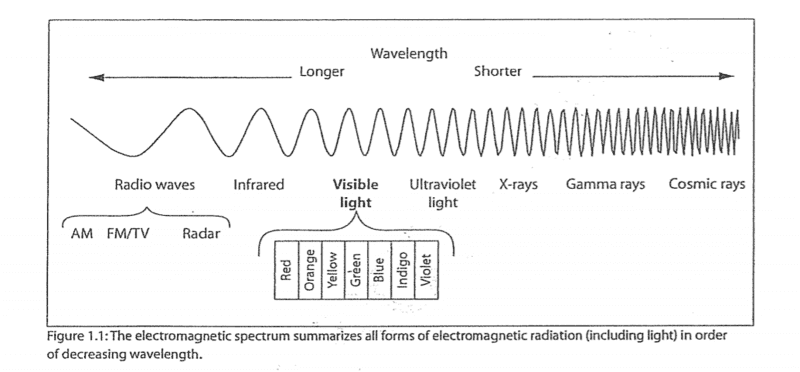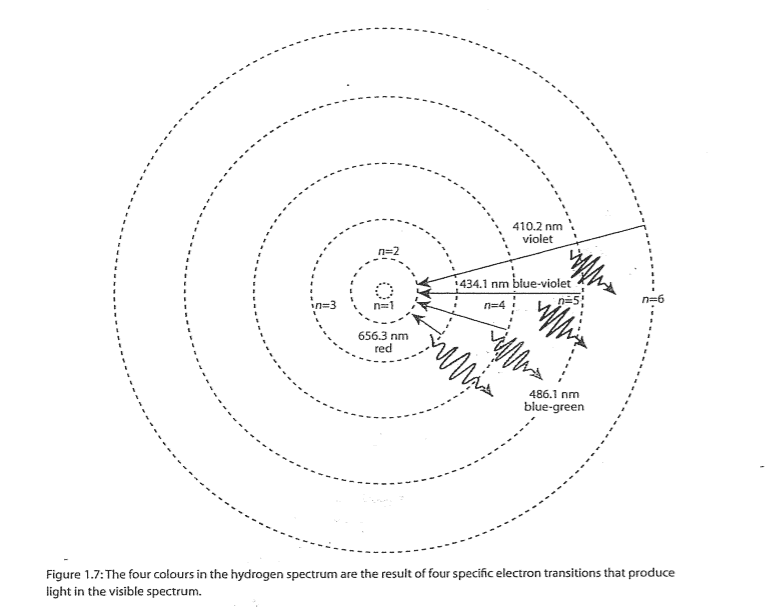# Emission Spectrum of an element

• Chemistry
• muissi97
muissi97
Homework Statement
The emission spectrum of an unknown element contains two lines-one in the visible portion of the spectrum, and the other, ultraviolet. Based on figure 1.1 and on what you have learned about Niels Bohr's model of the atom, account for the difference in energy between these lines.
Relevant Equations
The energy of a particle increases inverse to its wavelength, or in other words, particles with shorter wavelengths have higher energy.
When the electron absorbs energy from some external source, it jumps to a higher orbit or energy level. A "jump" from one level to another is called an electron transition. The attraction of the nucleus eventually pulls the electron back to a lower energy level. When it does, the energy that the electron absorbed is emitted. The energy emitted by the atom, according to Bohr, is the difference in energy between the two energy levels that the electron occupied. In the case of hydrogen, the four lines of the visible spectrum correspond to the electron transitions shown in Figure 1.7.The difference in energy between these two lines is that in the ultraviolet spectrum line, there is more energy because it has a shorter wavelength compared to the visible spectrum line as shown in figure 1.1 According to the Niels Bohr's model of the atom(figure 1.7) and figure 1.1, the least visible line with the shortest wavelength in the visible spectrum line is the violet line with a wavelength of 410.2 nm, this line carries the most energy in this spectrum, so the ultraviolet line must have a shorter wavelength than 410.2 nm for it to carry more energy than the visible spectrum. Also (in UV spectrum), the electron must jump to a higher energy level than the electron jump(transition) of the violet line.

Mentor
This question is so vague I am not sure it can be answered unambiguously.

At best you can tell what is the range of energies related to lines in the visible spectrum and what is the range of energies related to lines in the ultraviolet. That will let you calculate what is the range of possible differences between lines - regardless of the elements/substances involved.

It should be also doable to find out which of the all possible combinations of Z and initial/final energy levels fit in the range - but I won't be surprised if you will find out there is infinitely many such combinations.

EigenState137
Homework Statement:: The emission spectrum of an unknown element contains two lines-one in the visible portion of the spectrum, and the other, ultraviolet. Based on figure 1.1 and on what you have learned about Niels Bohr's model of the atom, account for the difference in energy between these lines.
Greetings,

What does the Bohr model state, mathematically, about the energy of some specific electron energy level?

What does the Bohr model state regarding a transition between energy levels?

What does the Rydberg equation state, mathematically, regarding two different emission transitions that terminate in the identical lower state?

From those considerations, what general conclusion can you draw regarding two emission transitions having different observed energies (wavelengths) that terminate in the identical lower state?

Best regards,
ES

•Astronuc and PeroK
Homework Helper
Gold Member
2022 Award
The wording in the question implies that the element has only 2 lines in its emission spectrum, which is impossible. So we must assume the question should be something like this:

"The emission spectrum of an unknown element contains many lines. Consider one line which is in the visible portion of the spectrum, and another line which is in the ultraviolet portion of the spectrum. Based on [blah blah] account for the difference in photon energy between these lines."

From the wording and the diagram of the EM spectrum, I would say no values/maths is needed – just a qualitative explanation.
_______________

•